# NCERT Solutions - Determinants, Exercise 4.4 JEE Notes | EduRev

## JEE : NCERT Solutions - Determinants, Exercise 4.4 JEE Notes | EduRev

The document NCERT Solutions - Determinants, Exercise 4.4 JEE Notes | EduRev is a part of the JEE Course Mathematics (Maths) Class 12.
All you need of JEE at this link: JEE

DETERMINANTS

Exercise 4.4

Question 1:

Write Minors and Cofactors of the elements of following determinants:
(i)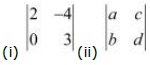(i) The given determinant is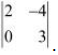Minor of element aij is Mij.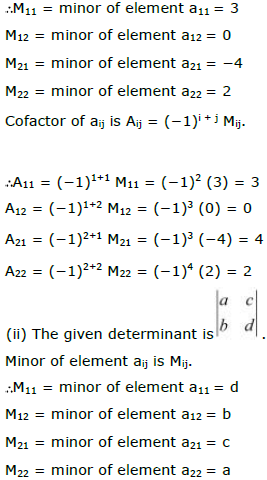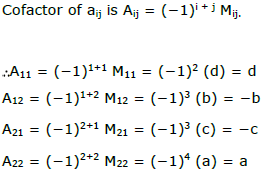Question 2: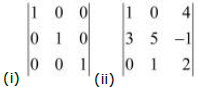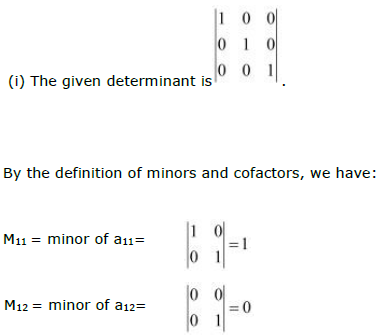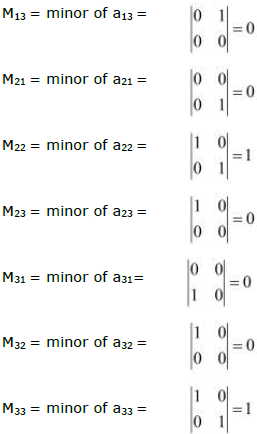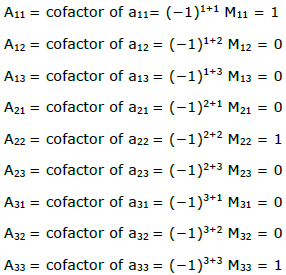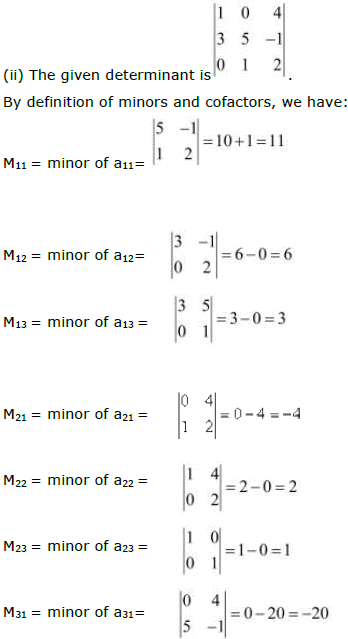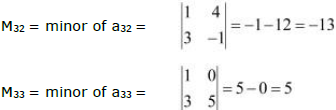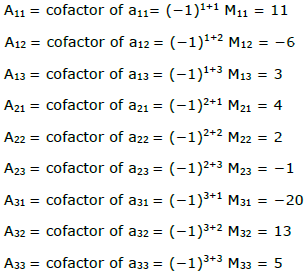Question 3: Using Cofactors of elements of second row, evaluate Δ =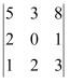.

The given determinant is.
We have: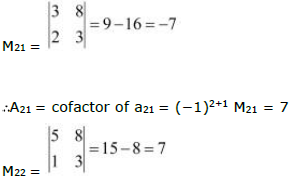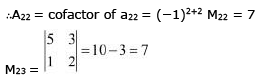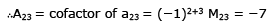We know that ∆ is equal to the sum of the product of the elements of the second row with their corresponding cofactors.

∆ = a21A21 + a22A22 + a23A23 = 2(7) + 0(7) + 1(−7) = 14 − 7 = 7

Question 4: Using Cofactors of elements of third column, evaluate Δ=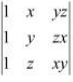The given determinant is.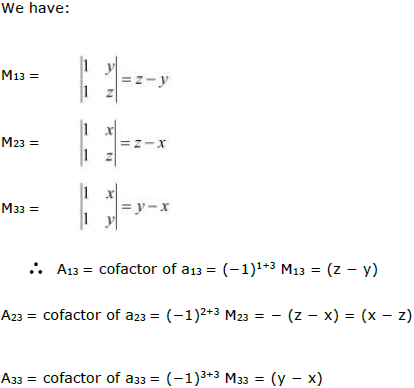We know that Δ is equal to the sum of the product of the elements of the second row
with their corresponding cofactors.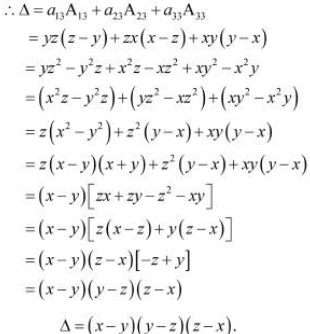Hence,

Question 5: For the matrices A and B, verify that (AB)′ = B'A' where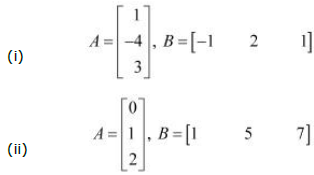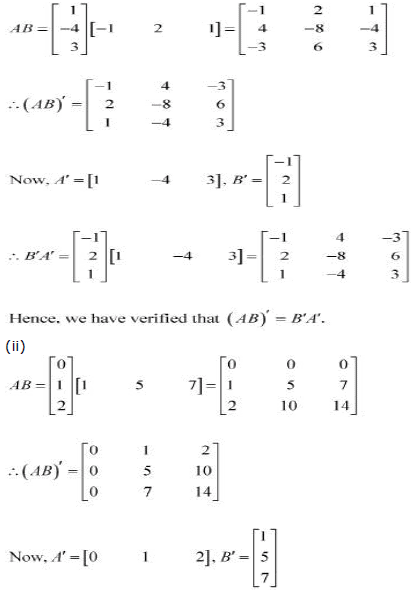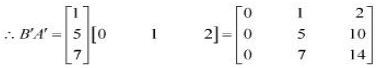Hence,

Offer running on EduRev: Apply code STAYHOME200 to get INR 200 off on our premium plan EduRev Infinity!

## Mathematics (Maths) Class 12

209 videos|222 docs|124 tests

,

,

,

,

,

,

,

,

,

,

,

,

,

,

,

,

,

,

,

,

,

,

,

,

;